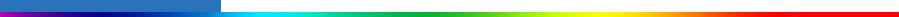Working Group I: The Scientific BasisGet Javascript Other reports in this collection

Appendix 11.1: Methods for projections of global-average sea level rise

This Appendix describes the methods used in this report to make sea level rise projections for the SRES scenarios for the 21st century. The results are discussed in Section 11.5.1.2 and shown in Figure 11.12 and Appendix II.

Global-average sea-level riseh(t) is a function of time t and is expressed relative to the level in 1990. It comprises several components, which are all zero at 1990: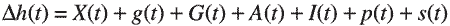The components are sea-level rise due to:

X   thermal expansion.
g    loss of mass of glaciers and ice caps.
G   loss of mass of the Greenland ice sheet due to projected and recent climate change.
A   loss of mass of the Antarctic ice sheet due to projected and recent climate change.
I     loss of mass of the Greenland and Antarctic ice sheets due to the ongoing adjustment to past climate change.
p    runoff from thawing of permafrost.
s    deposition of sediment on the ocean floor.

The components X, g, G and A are estimated for each of 35 SRES scenarios using the projections of an upwelling-diffusion energy-balance (UD/EB) model calibrated separately for each of seven AOGCMs (Appendix 9.1).

Thermal expansion X is obtained directly from the thermal expansion X m (t) projected by the UD/EB model: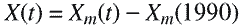No uncertainty is included in this term, because the uncertainty is sufficiently represented by the use of a range of AOGCMs. The term g from glaciers and ice caps is estimated using the global average temperature change Tm(t) projected by the UD/EB model. First, we obtain the loss of mass gu with respect to the glacier steady state without taking contraction of glacier area into account.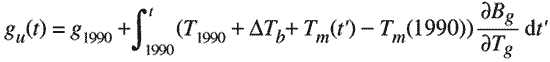where g1990 is the sea-level rise from glaciers and ice caps up to 1990 calculated from AOGCM results without contraction of glacier area, T1990 is the AOGCM global average temperature change at 1990 with respect to the climate of the late 19th century,Tb = 0.15 K the difference in the global average temperature between the late 19th century and the glacier steady state (see 11.5.1.1) and ðBgTg is the sensivity of global glacier mass balance for constant glacier area to global-average temperature change, expressed as sea level equivalent (from Table 11.11). Second, we estimate the loss of mass g s with respect to the glacier steady state taking into account contraction of glacier area. This is done by using an empirical relationship between the loss of mass for changing and for constant area. The relationship was obtained by a quadratic fit to the AOGCM IS92a results of Section 11.5.1.1.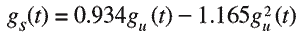for gu and gs in metres. Third, we calculate the change since 1990.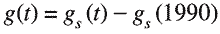The uncertainty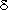g(t) on this term is calculated assuming an uncertainty of ±40% (standard deviation) in the mass balance sensitivities, as discussed in Section 11.5.1.1.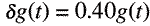The term G from the Greenland ice sheet is calculated according to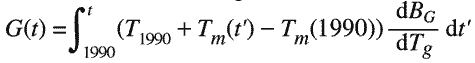where dBG/dTg is the sensitivity of the Greenland mass balance to global-average temperature change, expressed as sea level equivalent (from Table 11.12). The uncertainty on this term comprises two components, as discussed in Section 11.5.1.1. The first uncertainty is a mass balance uncertainty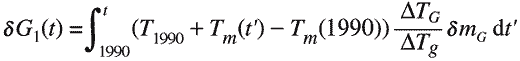wheremG = 0.05 mm/yr/°C andTG/Tg is the ratio of Greenland average temperature change to global average temperature change (from Table 11.12). The first uncertainty is the combination in quadrature of 0.03 mm/yr/°C from ablation parametrization, 0.03 mm/yr/°C from high-resolution patterns, and 0.02 mm/yr/°C from precipitation changes, as discussed in section 11.5.1.1. The second uncertainty is an ice-dynamic uncertainty.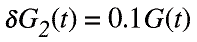The term A from the Antarctic ice sheet is calculated according to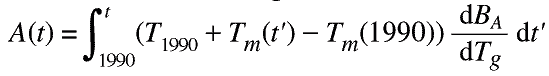where dBA/dTg is the sensitivity of the Antarctic mass balance to global-average temperature change, expressed as sea level equivalent (from Table 11.12). Ice-dynamical uncertainty for the Antarctic is not included and is discussed in Section 11.5.4.3. There is no uncertainty for ablation. Precipitation change uncertainty is calculated as discussed in Section 11.5.1.1 according to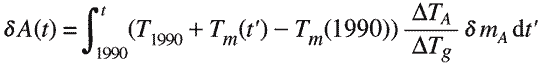wheremA = 0.08 mm/yr/°C andTA/Tg is the ratio of Antarctic average temperature change to global average temperature change (from Table 11.12).

The uncertainties on the above terms are combined in quadrature: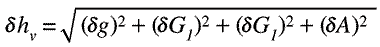The remaining terms are calculated assuming they contribute to sea-level rise at a constant rate, independent of AOGCM and scenario, thus:The rates each have a range of uncertainty. For dI/dt, this is 0.0 to 0.5 mm/yr (Section 11.3.1, Table 11.9), for dp/dt 0 to 0.23 mm/yr (the upper bound is more precisely 25 mm divided by 110 years, section 11.2.5), for ds/dt 0 to 0.05 mm/yr (Section 11.2.6, Table 11.9). The central rates are 0.25, 0.11 and 0.025 mm/yr for the three terms. We denote I calculated at the minimum rate by Imin and at the maximum rate by Imax; similarly for p and s. The minimum projected sea-level risehmin(t) for a given AOGCM and SRES scenario is given by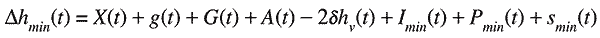and the maximum is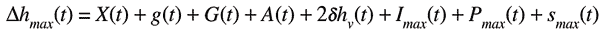In these formulae,hv has been doubled to convert from an uncertainty to a range, following Box 11.1.

 Table 11.17: Parameters used in sea-level projections to simulate AOGCM results.AOGCM T1990 (°C) g1990 (m) .Bg/.Tg (mm/yr/°C) dBG /dTg (mm/yr/°C) dBA/dTg (mm/yr/°C) .TG /Tg .TA/TgCSIRO Mk2 0.593 0.022 0.733 0.157 -0.373 2.042 1.120 CSM 1.3 0.567 0.021 0.608 0.146 -0.305 3.147 1.143 ECHAM4/OPYC3 0.780 0.027 0.608 0.029 -0.478 1.153 1.484 GFDL_R15_a 0.635 0.015 0.576 0.029 -0.177 1.879 0.799 HadCM2 0.603 0.027 0.613 0.096 -0.214 1.441 1.239 HadCM3 0.562 0.021 0.622 0.096 -0.354 1.443 1.288 DOE PCM 0.510 0.017 0.587 0.136 -0.484 2.165 1.618